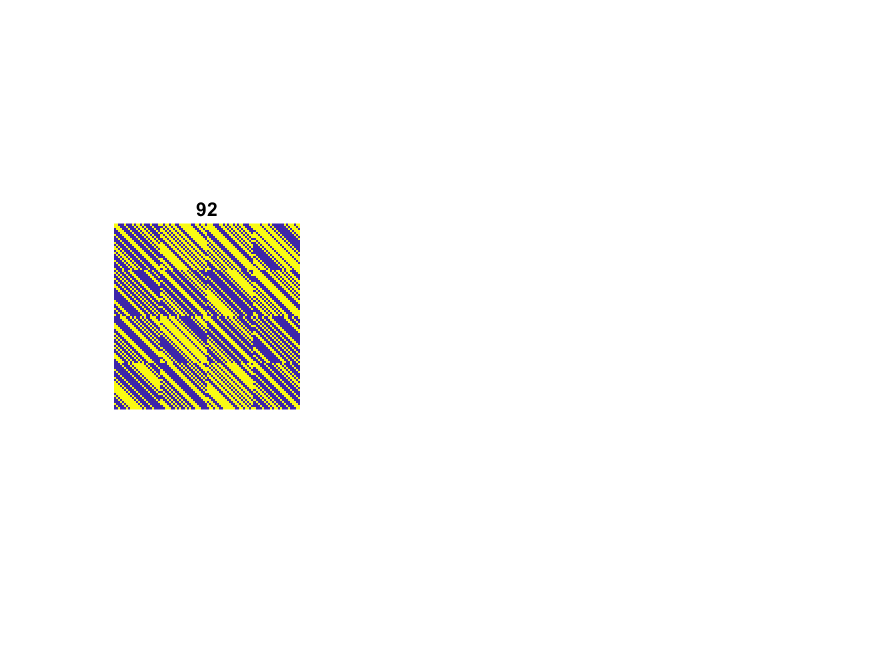## Cleve’s Corner: Cleve Moler on Mathematics and ComputingScientific computing, math & more

I have just returned from the ICIAM2019 conference in Valencia, Spain. It was a huge conference -- 4,000+ attendees, dozens of prize and invited talks, hundreds of parallel minisympsia. I gave a talk in a two-part minisymposium organized by Nick Higham and Rob Corless. I outlined the first part of the talk in this blog a month ago. This post outlines the second part, which was about Hadamard matrices. Some of it is taken from a post in this blog five years ago.

### Contents

The determinant of any matrix cannot be larger than the product of the length of its rows. This leads to Hadamard's inequality, for any $n$ by $n$ matrix, $A$, with elements +1 or -1,

$|\mbox{det}(A)| \le n^{n/2}$

A Hadamard Matrix is a square matrix $H$ with elements +1 or -1 whose rows (or columns) are mutually orthogonal, that is

$H^T H = nI$

A Hadamard matrix achieves equality in the Hadamard inequality. Conversely, any matrix achieving equality must be a Hadamard matrix.

$$H = \left( \begin{array}{rr} 1 & 1 \\ 1 & -1 \\ \end{array} \right)$$

Notice that

$|\mbox{det}(H)| = 2 = 2^{2/2}$

A direct search of all 3-by-3 matrices shows

$$A = \left( \begin{array}{rrr} 1 & 1 & 1 \\ 1 & -1 & 1 \\ 1 & 1 & -1 \end{array} \right)$$

and its permutations have the largest determinant.

But the rows of $A$ are not mutually orthogonal and

$|\mbox{det}(A)| = 4 < 3^{3/2} = 5.196$

We conclude no 3-by-3 Hadamard matrices exist.

$$H = \left( \begin{array}{rrrr} 1 & 1 & 1 & 1 \\ 1 & -1 & 1 & -1 \\ 1 & 1 & -1 & -1 \\ 1 & -1 & -1 & 1 \end{array} \right)$$

Notice

$|\mbox{det}(H)| = 16 = 4^{4/2}$

#### Existence

These three examples are special cases of this important fact: The order of a Hadamard matrix must be 1, 2, or a multiple of 4. But what about the reverse question, does a Hadamard matrix of order $n = 4k$ exist for every $k$? Nobody knows for sure. This is an important open mathematical problem.

#### Generation

It is easy to create Hadamard matrices of some sizes. If $H$ is any Hadamard matrix, then so is the block matrix

$$\left( \begin{array}{rr} H & H \\ H & -H \\ \end{array} \right)$$

You can start a recursion with the 1-by-1 matrix $H = 1$ and generate Hadamard matrices whose orders are powers of two. The MATLAB function hadamard can also start with a 12-by-12 or a 20-by-20 $H$. Here is what you get. Yellow is +1 and blue is -1.#### Baumert92

I was present at a milestone in the history of Hadamard matrices, By 1961 it was known how to construct Hadamard matrices for all orders $n \le 100$ that are multiples of four, except $n = 92$. In 1961 I had a summer job at JPL, Caltech's Jet Propulsion Lab. My office was in a temporary trailer and my trailer mate was an algebraist named Len Baumert. Len proudly showed me a color graphic that he had just made with an hour-long computation on JPL's main-frame and that he was proposing for the cover of Scientific American. It was a 92-by-92 matrix made up from 23-by-23 blocks of alternating light and dark cells representing +1 and -1. The graphic didn't make the cover of Scientific American, but I kept my copy for a long time.

Len had filled in the last value of $n$ less than 100. His paper with his colleagues Sol Golomb, a professor at USC, and Marshall Hall, Jr., a professor at Caltech, was published in the prestigious Bulletin of the AMS. It turns out that I had taken a course on difference sets, the mathematics involved in generating the matrix, from Hall at Caltech.

Here is a MATLAB picture and code for Baumert92.#### References

Leonard Baumert, S. W. Golomb, and Marshall Hall, Jr, "Discovery of an Hadamard Matrix of Order 92", <http://dx.doi.org/10.1090%2FS0002-9904-1962-10761-7>, Bulletin of the American Mathematical Society 68 (1962), 237-238.

#### code

   type Baumert92.m

function H = Baumert92
% Baumert92  Hadamard matrix of order 92.
% H = Baumert92

% Baumert, Golomb, and Hall, Bulletin AMS 68, 1962, 237-238.
% http://dx.doi.org/10.1090%2FS0002-9904-1962-10761-7

% First row of 23-by-23 circulants.

M = [ '+ + - - - + - - - + - + + - + - - - + - - - +'
'+ - + + - + + - - + + + + + + - - + + - + + -'
'+ + + - - - + + - + - + + - + - + + - - - + +'
'+ + + - + + + - + - - - - - - + - + + + - + +' ];

M = 2*(M(:,1:2:end)=='+')-1;

A = toeplitz([1 M(1,end:-1:2)],M(1,:));
B = toeplitz([1 M(2,end:-1:2)],M(2,:));
C = toeplitz([1 M(3,end:-1:2)],M(3,:));
D = toeplitz([1 M(4,end:-1:2)],M(4,:));

H = [A B C D; -B A -D C; -C D A -B; -D -C B A];


Published with MATLAB® R2018b

|